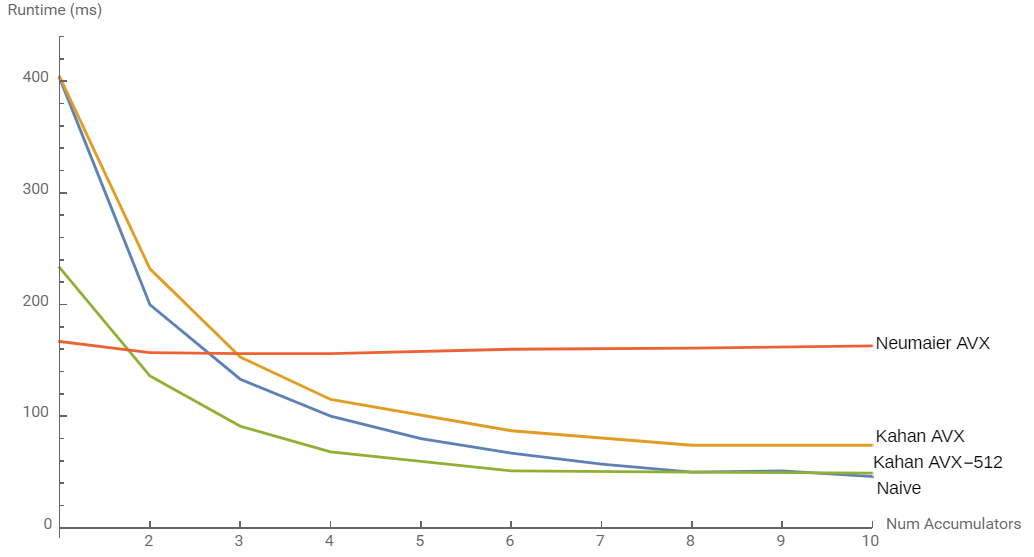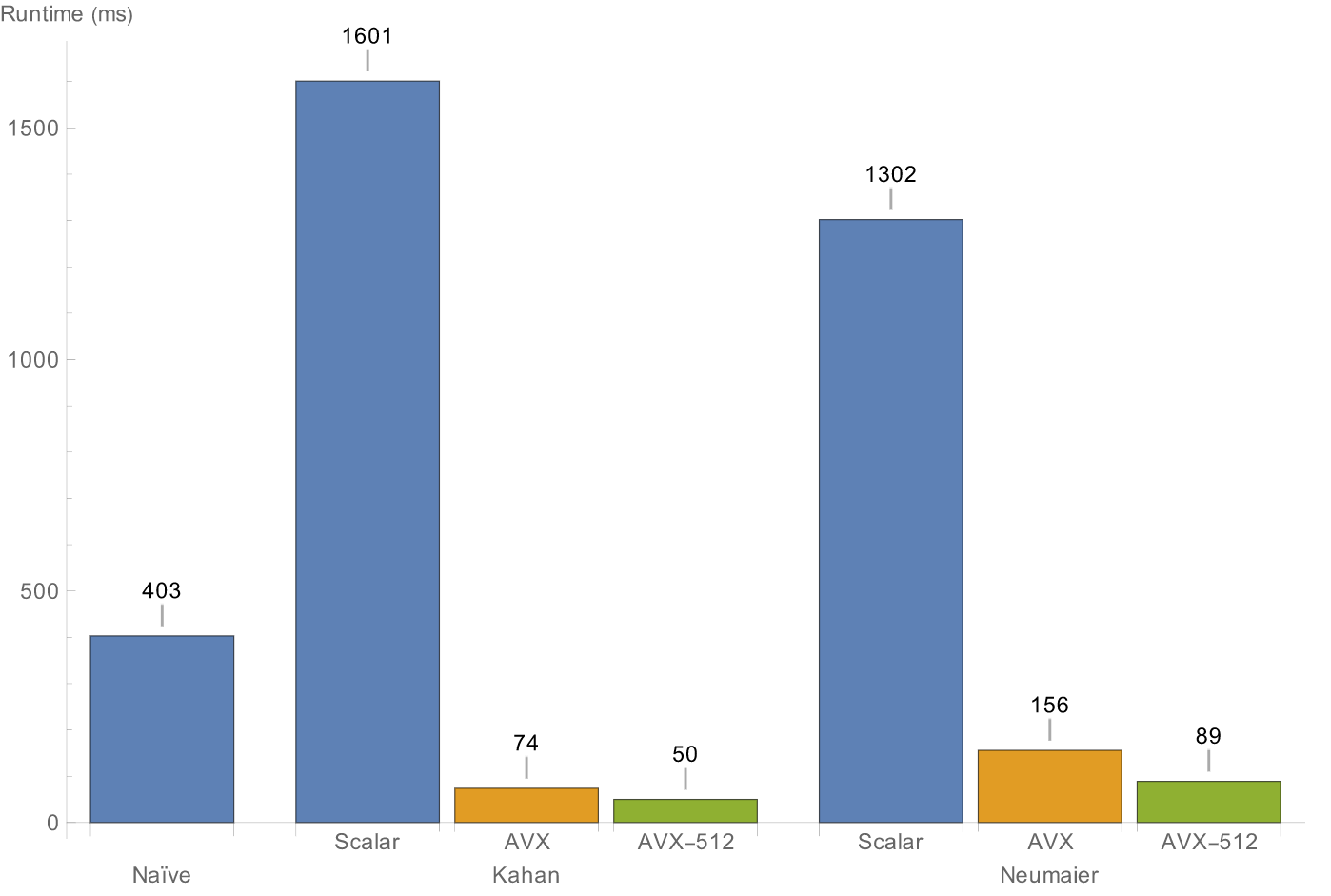Let's say you need to sum a large array of floating-point numbers, maybe because you're calculating the arithmetic mean or variance. This post shows how to do so accurately and quickly.

Here's what the accuracy-naïve, performance-naïve implementation looks like (Godbolt):

#### Improving Accuracy

When summing floating-point numbers, we need to consider the following:

• Overflow Adding two numbers that have the value `FLT_MAX` (maximum value of `float`, ~3.4e38) using a single-precision `float` accumulator will obviously overflow. To work around this, we can simply use a double-precision accumulator and be able to safely sum `DBL_MAX ÷ FLT_MAX ≈ 1.8e308 ÷ 3.4e38 ≈ 5.2e269` floats. That's a lot—more than the max `uint64_t`.

(If you're summing a huge number of huge double-precision numbers, using `long double` is sometimes a possibility. On x86 that usually uses the legacy 80-bit x87 float-point unit, which is incapable of SIMD operations and has half the throughput of the SSE FPU on recent Intel and AMD CPUs.)

• Floating-point error Adding two numbers of very different magnitude causes loss of significant digits. Using a double-precision accumulator helps, but we can also use compensated summation to limit the error to be essentially independent of the number of values we're adding. Kahan summation is the most famous algorithm; it's simple and effective. Neumaier's improved version ("Kahan-Babuška Algorithm") is correct in some cases where Kahan is not, but is significantly slower. Pairwise summation is also popular and requires the fewest operations, but has somewhat worse error and needs to be implemented with careful concern for data locality (cache-awareness).

Here's a (more) accurate implementation that uses Kahan summation (Godbolt):

#### Improving Speed

We want to sum the numbers quickly. Here's what we'll do:

• Break the loop-carried dependency. Modern CPUs can start a new operation before the previous one completes. This is called instruction pipelining. On Intel's Skylake architecture, two additions can be started per cycle ("throughput") despite taking four cycles to complete ("latency"). The equivalent numbers for AMD's Ryzen are one and three.

For this to happen, the second operation must not depend on the first operation's result. In the performance-naïve code above, each loop iteration depends on the previous iteration's result (there's a "loop-carried dependency"). By using multiple accumulators in the main loop that we sum together later, we break this dependency chain. The benefits of this are visible in scalar and SIMD code (below), and even in a JavaScript implementation run in v8. Notice though that it has minimal effect on Neumaier's algorithm because it requires so many more operations that there is minimal room for pipelining of loop iterations.Effect of varying numbers of accumulators when summing a 187,500-element array of floats (fits in L2 cache) 2000 times. Run on an Intel Xeon Cascade Lake CPU (3.1GHz base). Compiled with GCC 8.1, except "naïve", which was compiled with Clang 9.0 to avoid a bad optimization with 8 accumulators in GCC. This also effectively unrolls the loop, which itself can improve performance.

• Use SIMD. Kahan and Neumaier summation can be trivially parallelized to operate on four (AVX) or eight (AVX-512) doubles at a time.

Clang with `-ffast-math` (which allows reordering of floating-point operations) does both of these optimizations automatically, although it only uses four vectors of accumulators (not quite enough for max speed). GCC auto-vectorizes but doesn't unroll or use multiple accumulators. However, `-ffast-math` is unsafe to use with compensated summation because the compiler can and does remove operations that are algebrically void.

Below are the benchmark results. We get a final speed up of ~8x over the naïve version (along with better floating-point accuracy) and a ~32x speedup over scalar by optimizing the Kahan algorithm.Comparison of the different summation methods (same setup as above).

Here are the final AVX(+BMI2) and AVX-512 implementations. (This code uses Intel intrinsics, which are documented here.)

The actual code used to benchmark (harder to read but easier to tinker with) is located here. It includes AVX and AVX-512 Neumaier implementations not shown above.

If you have any comments, feel free to email or tweet using the links in the footer, or open an issue in my GitHub pages repo. Corrections and improvements greatly appreciated.